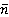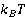### Create an Account

Home / Questions / Obtain an equation for the mean quantum number of an Einstem oscillator as a function of ...

# Obtain an equation for the mean quantum number of an Einstem oscillator as a function of the temperature Calculate for

Obtain an equation for the mean quantum numberof an Einstem oscillator as a function of the temperature. Calculatefor/hω0 = 0, 1, 2, 3, 4, 10, 50, 100 (ignore the physical reality of melting of the crystal!).

Jun 09 2020 View more View LessSubscribe To Get Solution